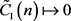﻿ 黎曼流形上Laplace算子的高阶特征值下界估计

# 黎曼流形上Laplace算子的高阶特征值下界估计Lower Bound Estimation of Higher Eigenvalues of Laplace Operators on Riemannian Manifold

Abstract: In this paper, we study the lower bound estimates of higher eigenvalues of Lapalce operator on Riemannian manifold. For Riemannian manifolds with negative lower bounds of Ricci curvature, Li-Yau obtained qualitative lower bound estimates. In this paper, we use the method of the gradient estimation of gradient function of the hot kernel and Harnack type inequality; we give the quantitative lower bound estimation of higher eigenvalues on Riemannian manifold with negative lower bound of Ricci curvature.

1. 引言以及主要结果

$\left(\Delta -\frac{\partial }{\partial t}\right)u\left(x,t\right)=0$ ，在 $M×\left[0,\infty \right)$(1.1)

$F\left(x,t\right)={|\nabla f|}^{2}-\alpha \left(t\right){f}_{t}$$\alpha \left(t\right)\in \left[0,T\right]$

$F\left(x,t\right)=t\left({|\nabla f|}^{2}-\alpha {f}_{t}\right)$

${\lambda }_{1}\ge \frac{{\text{π}}^{2}}{{d}^{2}}-\frac{15}{16}K$(1.2)

${\lambda }_{1}\ge \frac{{\text{π}}^{2}}{2{d}^{2}}{\left(\frac{1}{4}{C}_{n}\sqrt{K{d}^{2}}\right)}^{2}{\left[\mathrm{exp}\left(\frac{1}{4}{C}_{n}\sqrt{K{d}^{2}}\right)-1\right]}^{-2}$(1.3)

$\left\{\begin{array}{l}n\le 7,K\ge 4\left(n-1\right){H}_{0}^{2},\\ 3

${\lambda }_{1}\ge \frac{{\text{π}}^{2}}{4{\rho }^{2}}{\left[\frac{1}{2}\sqrt{\left(n-1\right)R{\rho }^{2}}\right]}^{2}{\left[\mathrm{exp}\left(\frac{1}{2}\sqrt{\left(n-1\right)R{\rho }^{2}}\right)-1\right]}^{-2}$(1.4)

${\lambda }_{k}\ge {C}_{1}\left(n\right)\frac{{k}^{2/n}{\text{π}}^{2}}{{d}^{2}}\mathrm{exp}\left(-{\stackrel{˜}{C}}_{1}\left(n\right)\sqrt{K{d}^{2}}\right)-\frac{15}{16}K$(1.5)

${C}_{1}\left(n\right)=\left(\frac{15}{8n}+1\right)\mathrm{exp}\left(-2\sqrt{\frac{16{\text{π}}^{2}}{15n}}\right)$${\stackrel{˜}{C}}_{1}\left(n\right)=\frac{2}{\sqrt{n-1}}$

${\lambda }_{k}\ge \frac{{k}^{2/n}{\text{π}}^{2}}{{d}^{2}}-\delta \cdot K$

${\lambda }_{k}\ge {C}_{2}\left(n\right)\frac{{k}^{2/n}{\pi }^{2}}{{d}^{2}}\mathrm{exp}\left(-{\stackrel{˜}{C}}_{2}\left(n\right)\sqrt{K{d}^{2}}\right)$(1.6)

${C}_{2}\left(n\right)=\frac{{\text{π}}^{2}}{15}{\left(\frac{15}{8n}+1\right)}^{2}\mathrm{exp}\left(-2\sqrt{\frac{16{\text{π}}^{2}}{15n}}\right)$${\stackrel{˜}{C}}_{2}\left(n\right)=\frac{2}{\sqrt{n-1}}+\frac{\sqrt{2}}{2}$

${\lambda }_{k}\ge {C}_{3}\left(n\right)\frac{{k}^{2/n}{\pi }^{2}}{{d}^{2}}\mathrm{exp}\left(-{\stackrel{˜}{C}}_{3}\left(n\right)\sqrt{K{d}^{2}}\right)$(1.7)

${C}_{3}\left(n\right)={\left(\frac{15}{8n}+1\right)}^{-2}\mathrm{exp}\left(-2\sqrt{\frac{16{\text{π}}^{2}}{15n}}\right)$${\stackrel{˜}{C}}_{3}\left(n\right)=\frac{2}{\sqrt{n-1}}+\frac{\sqrt{n-1}}{2}$

$n\to +\infty$ 时， ${C}_{3}\left(n\right)↦1$ ，此时就有

${\lambda }_{k}\ge \frac{{k}^{2/n}{\text{π}}^{2}}{{d}^{2}}\mathrm{exp}\left(-{C}_{n}\sqrt{K{d}^{2}}\right)$

${C}_{n}$ 仅依赖于常数n。

${\lambda }_{k}\ge {C}_{4}\left(n\right)\frac{{k}^{2/n}{\text{π}}^{2}}{{d}^{2}}\mathrm{exp}\left(-{\stackrel{˜}{C}}_{4}\left(n\right)\sqrt{K}\right)$(1.8)

${C}_{4}={\left(\frac{15}{8n}+1\right)}^{-2}\mathrm{exp}\left(-2\sqrt{\frac{16{\text{π}}^{2}}{15n}}\right)$${\stackrel{˜}{C}}_{4}=\frac{2d}{\sqrt{n-1}}+\sqrt{\left(n-1\right){\rho }^{2}}$

2. Harnack不等式

$\left(\Delta -\frac{\partial }{\partial t}\right)f=-{|\nabla f|}^{2}$(2.1)

$F\left(x,t\right)=t\left({|\nabla f|}^{2}-\alpha {f}_{t}\right)$ ，对此，Schoen-Yau  得到了如下梯度估计

${|\nabla f|}^{2}-\alpha {f}_{t}\le \frac{n{\alpha }^{2}}{2t}+\frac{n{\alpha }^{2}K}{2\left(\alpha -1\right)}$$t>0$$\alpha >1$(2.2)

${|\nabla f|}^{2}-\alpha {f}_{t}\le \frac{n{\alpha }^{2}}{2t}+\frac{n{\alpha }^{2}K}{4\left(\alpha -1\right)}$$t>0$$\alpha >1$(2.3)

$u\left({x}_{1},{t}_{1}\right)\le u\left({x}_{2},{t}_{2}\right){\left(\frac{{t}_{2}}{{t}_{1}}\right)}^{n\alpha /2}\mathrm{exp}\left[\frac{\alpha {\rho }^{2}}{4\left({t}_{2}-{t}_{1}\right)}+\frac{n\alpha K\left({t}_{2}-{t}_{1}\right)}{4\left(\alpha -1\right)}\right]$(2.4)

$f=\mathrm{log}u\left(x,t\right)$ ，则

$f\left({x}_{1},{t}_{1}\right)-f\left({x}_{2},{t}_{2}\right)=f\left[\eta \left(1\right)\right]-f\left[\eta \left(0\right)\right]={f\left[\eta \left(s\right)\right]|}_{s=1}-{f\left[\eta \left(s\right)\right]|}_{s=0}$

$\begin{array}{c}f\left({x}_{1},{t}_{1}\right)-f\left({x}_{2},{t}_{2}\right)={\int }_{0}^{1}\frac{\text{d}f\left[\eta \left(s\right)\right]}{\text{d}s}\text{d}s={\int }_{0}^{1}\left[〈\gamma ,\nabla f〉-\left({t}_{2}-{t}_{1}\right){f}_{t}\right]\text{d}s\\ \le {\int }_{0}^{1}\left[|\stackrel{˙}{\gamma }||\nabla f|-\left({t}_{2}-{t}_{1}\right){f}_{t}\right]\text{d}s\end{array}$(2.5)

$-{f}_{t}\le \frac{1}{\alpha }\left(\frac{n{\alpha }^{2}}{2t}+\frac{n{\alpha }^{2}K}{4\left(\alpha -1\right)}-{|\nabla f|}^{2}\right)$(2.6)

$\begin{array}{c}f\left({x}_{1},{t}_{1}\right)-f\left({x}_{2},{t}_{2}\right)\le {\int }_{0}^{1}\left[\sigma |\nabla f|+\frac{{t}_{2}-{t}_{1}}{\alpha }\left(\frac{n\alpha {}^{2}}{2t}+\frac{n{\alpha }^{2}K}{4\left(\alpha -1\right)}-{|\nabla f|}^{2}\right)\right]\text{d}s\\ ={\int }_{0}^{1}\left[-\frac{{t}_{2}-{t}_{1}}{\alpha }{|\nabla f|}^{2}+\sigma |\nabla f|+\frac{{t}_{2}-{t}_{1}}{\alpha }\left(\frac{n\alpha {}^{2}}{2t}+\frac{n{\alpha }^{2}K}{4\left(\alpha -1\right)}\right)\right]\text{d}s\end{array}$

$\frac{\alpha {\sigma }^{2}}{4\left({t}_{2}-{t}_{1}\right)}+\frac{{t}_{2}-{t}_{1}}{\alpha }\left[\frac{n\alpha {}^{2}}{2t}+\frac{n{\alpha }^{2}K}{4\left(\alpha -1\right)}\right]$(2.7)

$t=\left(1-s\right){t}_{2}+s{t}_{1}$ ，直接由(2.7)式得到

$\begin{array}{c}f\left({x}_{1},{t}_{1}\right)-f\left({x}_{2},{t}_{2}\right)\le \frac{\alpha {\sigma }^{2}}{4\left({t}_{2}-{t}_{1}\right)}+\frac{n\alpha K\left({t}_{2}-{t}_{1}\right)}{4\left(\alpha -1\right)}+\frac{n\alpha \left({t}_{2}-{t}_{1}\right)}{2}{\int }_{0}^{1}\frac{\text{d}s}{\left(1-s\right){t}_{2}+s{t}_{1}}\\ =\frac{\alpha {\sigma }^{2}}{4\left({t}_{2}-{t}_{1}\right)}+\frac{n\alpha K\left({t}_{2}-{t}_{1}\right)}{4\left(\alpha -1\right)}+\frac{n\alpha }{2}\mathrm{ln}\left(\frac{{t}_{2}}{{t}_{1}}\right)\end{array}$ (2.8)

(2.8)式不等式两边同时取指数函数exp，就可以得到(2.4)式。特别地，取 $\alpha =2$ 时，(2.4)式就可以得到

$u\left({x}_{1},{t}_{1}\right)\le u\left({x}_{2},{t}_{2}\right){\left(\frac{{t}_{2}}{{t}_{1}}\right)}^{n}\mathrm{exp}\left[\frac{{\sigma }^{2}}{2\left({t}_{2}-{t}_{1}\right)}+\frac{nK\left({t}_{2}-{t}_{1}\right)}{2}\right]$(2.9)

3. 主要定理的证明

${V}_{x}\left(r\right)\ge {\left(\frac{\mathrm{sinh}\left(\sqrt{\frac{K{r}^{2}}{n-1}}\right)}{\mathrm{sinh}\left(\sqrt{\frac{K{d}^{2}}{n-1}}\right)}\right)}^{n}{V}_{x}\left(d\right)$(3.1)

$H\left(x,x,{t}_{1}\right)\le H\left(x,y,{t}_{1}\right){\left(\frac{{t}_{2}}{{t}_{1}}\right)}^{n}\mathrm{exp}\left[\frac{{\rho }^{2}}{2\left({t}_{2}-{t}_{1}\right)}+\frac{nK\left({t}_{2}-{t}_{1}\right)}{2}\right]$

${t}_{1}=t$${t}_{2}-{t}_{1}=\beta t$ ，移项并对y作积分，因为 ${\int }_{M}H\left(x,y,t\right)\text{d}y=1$ ，因此有

$H\left(x,x,t\right){\int }_{M}\mathrm{exp}\left(-\frac{{\sigma }^{2}}{2\beta t}\right)\text{d}y\le {\left(\beta +1\right)}^{n}\mathrm{exp}\left(\frac{nK\beta t}{2}\right)$(3.2)

${\int }_{M}\mathrm{exp}\left(-\frac{{\sigma }^{2}}{2\beta t}\right)\text{d}y\ge {\left(\frac{\mathrm{sinh}\left(\sqrt{\frac{K{r}^{2}}{n-1}}\right)}{\mathrm{sinh}\left(\sqrt{\frac{K{d}^{2}}{n-1}}\right)}\right)}^{n}{V}_{x}\left(d\right)\mathrm{exp}\left(-\frac{{r}^{2}}{2\beta t}\right)\ge \frac{{r}^{n}{V}_{x}\left(d\right)}{{d}^{n}\mathrm{exp}\left(n\sqrt{\frac{K{d}^{2}}{n-1}}\right)}\mathrm{exp}\left(-\frac{{r}^{2}}{2\beta t}\right)$

${\int }_{M}H\left(x,x,t\right)\text{d}x=\underset{i=0}{\overset{\infty }{\sum }}\mathrm{exp}\left(-{\lambda }_{i}t\right)\ge k\mathrm{exp}\left(-{\lambda }_{k}t\right)$

$\frac{k}{{\left(\beta +1\right)}^{n}{d}^{n}\mathrm{exp}\left(n\sqrt{\frac{K{d}^{2}}{n-1}}\right)}\le {r}^{-n}\mathrm{exp}\left[\frac{{r}^{2}}{2\beta t}+\left({\lambda }_{k}+\frac{nK\beta }{2}\right)t\right]$(3.3)

$\frac{n\beta }{2}=\frac{15}{16}$ ，则 $\beta =\frac{15}{8n}$ 。假设r是确定的，对t取极小值，(3.3)式可得

$\frac{k}{{\left(\frac{15}{8n}+1\right)}^{n}{d}^{n}\mathrm{exp}\left(n\sqrt{\frac{K{d}^{2}}{n-1}}\right)}\le {r}^{-n}\mathrm{exp}\left[\sqrt{\frac{16n{r}^{2}}{15}\left({\lambda }_{k}+\frac{15}{16}K\right)}\right]$(3.4)

${r}^{2}\left({\lambda }_{k}+\frac{15}{16}K\right)={\text{π}}^{2}$ ，从而有

$\frac{k{\text{π}}^{n}}{{\left(\frac{15}{8n}+1\right)}^{n}{d}^{n}\mathrm{exp}\left(n\sqrt{\frac{K{d}^{2}}{n-1}}+\sqrt{\frac{16n{\text{π}}^{2}}{15}}\right)}\le {\left({\lambda }_{k}+\frac{15}{16}K\right)}^{n/2}$(3.5)

${\lambda }_{k}\ge \frac{{k}^{2/n}{\text{π}}^{2}}{{\left(\frac{15}{8n}+1\right)}^{2}{d}^{2}\mathrm{exp}\left(2\sqrt{\frac{K{d}^{2}}{n-1}}\right)\mathrm{exp}\left(2\sqrt{\frac{16{\text{π}}^{2}}{15n}}\right)}-\frac{15}{16}K$

${C}_{1}\left(n\right)={\left(\frac{15}{8n}+1\right)}^{-2}\mathrm{exp}\left(-2\sqrt{\frac{16{\text{π}}^{2}}{15n}}\right)$${\stackrel{˜}{C}}_{1}\left(n\right)=\frac{2}{\sqrt{n-1}}$

$n↦\infty$ 时， ${C}_{1}\left(n\right)↦1$，(1.5)式可表示为

${\lambda }_{k}\ge \frac{{k}^{2/n}{\text{π}}^{2}}{{d}^{2}}-\frac{15}{16}K$

${\lambda }_{k}\ge \frac{{k}^{2/n}{\text{π}}^{2}}{{d}^{2}}-\delta \cdot K$

${\lambda }_{1}\ge \frac{{\text{π}}^{2}}{2{d}^{2}}{\left(\frac{1}{4}\sqrt{2K{d}^{2}}\right)}^{2}{\left[\mathrm{exp}\left(\frac{1}{4}\sqrt{2K{d}^{2}}\right)-1\right]}^{-2}$

$\frac{K}{{\lambda }_{1}}\le \frac{16}{{\text{π}}^{2}}{\left[\mathrm{exp}\left(\frac{1}{4}\sqrt{2K{d}^{2}}\right)-1\right]}^{2}$(3.6)

$\begin{array}{c}{\left({\lambda }_{k}+\frac{15}{16}K\right)}^{n/2}={\lambda }_{k}^{n/2}{\left(1+\frac{15K}{16{\lambda }_{k}}\right)}^{n/2}\le {\lambda }_{k}^{n/2}{\left(1+\frac{15K}{16{\lambda }_{1}}\right)}^{n/2}\\ \le {\lambda }_{k}^{n/2}{\left(1+\frac{15}{{\text{π}}^{2}}{\left[\mathrm{exp}\left(\frac{1}{4}\sqrt{2K{d}^{2}}\right)-1\right]}^{2}\right)}^{n/2}\\ \le {\lambda }_{k}^{n/2}{\left(\frac{15}{{\text{π}}^{2}}\right)}^{n/2}\mathrm{exp}\left(\frac{n}{4}\sqrt{2K{d}^{2}}\right)\end{array}$

${\lambda }_{k}\ge \frac{{k}^{2/n}{\text{π}}^{2}}{\frac{15}{{\text{π}}^{2}}{\left(\frac{15}{8n}+1\right)}^{2}{d}^{2}\mathrm{exp}\left(2\sqrt{\frac{K{d}^{2}}{n-1}}\right)\mathrm{exp}\left(2\sqrt{\frac{16{\text{π}}^{2}}{15n}}\right)}\mathrm{exp}\left(-\frac{1}{2}\sqrt{2K{d}^{2}}\right)$(3.7)

${C}_{2}\left(n\right)=\frac{{\text{π}}^{2}}{15}{\left(\frac{15}{8n}+1\right)}^{-2}\mathrm{exp}\left(-2\sqrt{\frac{16{\text{π}}^{2}}{15n}}\right)$${\stackrel{˜}{C}}_{2}\left(n\right)=\frac{2}{\sqrt{n-1}}+\frac{\sqrt{2}}{2}$

$n\ge 3$ 时， ${C}_{n}=\sqrt{n-1}$ ，直接由(1.3)式可等价于

${\lambda }_{1}\ge \frac{{\text{π}}^{2}}{{d}^{2}}{\left(\frac{1}{4}\sqrt{\left(n-1\right)K{d}^{2}}\right)}^{2}{\left[\mathrm{exp}\left(\frac{1}{4}\sqrt{\left(n-1\right)K{d}^{2}}\right)-1\right]}^{-2}$(3.8)

$1+\sqrt{\frac{\left(n-1\right){\text{π}}^{2}}{16}\frac{K}{{\lambda }_{1}}}\le \mathrm{exp}\left(\frac{1}{4}\sqrt{\left(n-1\right)K{d}^{2}}\right)$(3.9)

$\begin{array}{c}{\left({\lambda }_{k}+\frac{15}{16}K\right)}^{n/2}={\lambda }_{k}^{n/2}{\left(1+\frac{15K}{16{\lambda }_{k}}\right)}^{n/2}\le {\lambda }_{k}^{n/2}{\left(1+\frac{15K}{16{\lambda }_{1}}\right)}^{n/2}\\ \le {\lambda }_{k}^{n/2}{\left(1+{\left[\mathrm{exp}\left(\frac{1}{4}\sqrt{\left(n-1\right)K{d}^{2}}\right)-1\right]}^{2}\right)}^{n/2}\\ \le {\lambda }_{k}^{n/2}\mathrm{exp}\left(\frac{n}{4}\sqrt{\left(n-1\right)K{d}^{2}}\right)\end{array}$

${\lambda }_{k}\ge \frac{{k}^{2/n}{\text{π}}^{2}}{{\left(\frac{15}{8n}+1\right)}^{2}{d}^{2}\mathrm{exp}\left(2\sqrt{\frac{K{d}^{2}}{n-1}}\right)\mathrm{exp}\left(2\sqrt{\frac{16{\text{π}}^{2}}{15n}}\right)}\mathrm{exp}\left(-\frac{1}{2}\sqrt{\left(n-1\right)K{d}^{2}}\right)$

${C}_{3}\left(n\right)={\left(\frac{15}{8n}+1\right)}^{-2}\mathrm{exp}\left(-2\sqrt{\frac{16{\text{π}}^{2}}{15n}}\right)$${\stackrel{˜}{C}}_{3}\left(n\right)=\frac{2}{\sqrt{n-1}}+\frac{\sqrt{n-1}}{2}$

${\lambda }_{k}\ge \frac{{k}^{2/n}{\text{π}}^{2}}{{d}^{2}}\mathrm{exp}\left(-{C}_{n}\sqrt{K{d}^{2}}\right)$

${C}_{n}$ 仅依赖于常数n，证毕。

4. 结束语

NOTES

*通讯作者。

 Li, P. and Yau, S. (1986) On the Parabolic Kernel of the Schrödinger Operator. Acta Mathematica, 156, 153-201.
https://doi.org/10.1007/BF02399203

 孙和军. 紧Riemann流形的高阶特征值估计[J]. 数学学报, 2006, 49(3): 539-548.

 蔡开仁. 紧致黎曼流形的第一特征值[J]. 杭州师范大学学报(社会科学版), 2000(3): 1-6.

 贾方. Ricci曲率具有负下界的紧致Riemann流形第一特征值的估计[J]. 数学年刊(中文版), 1991(4): 496-502.

 杨洪苍. 带边紧致黎曼流形Riemann边界条件的第一特征值估计[J]. 数学学报, 1991, 34(3): 329-342.

 Schoen, R. and Yau, S.T. (1994) Lectures on Differential Geometry. International Press, Boston, MA.

 Davies, E. (1989) Heat Kernels and Spectral Theory. Cambridge University Press, Cambridge.
https://doi.org/10.1017/CBO9780511566158

Top